## MTSO September News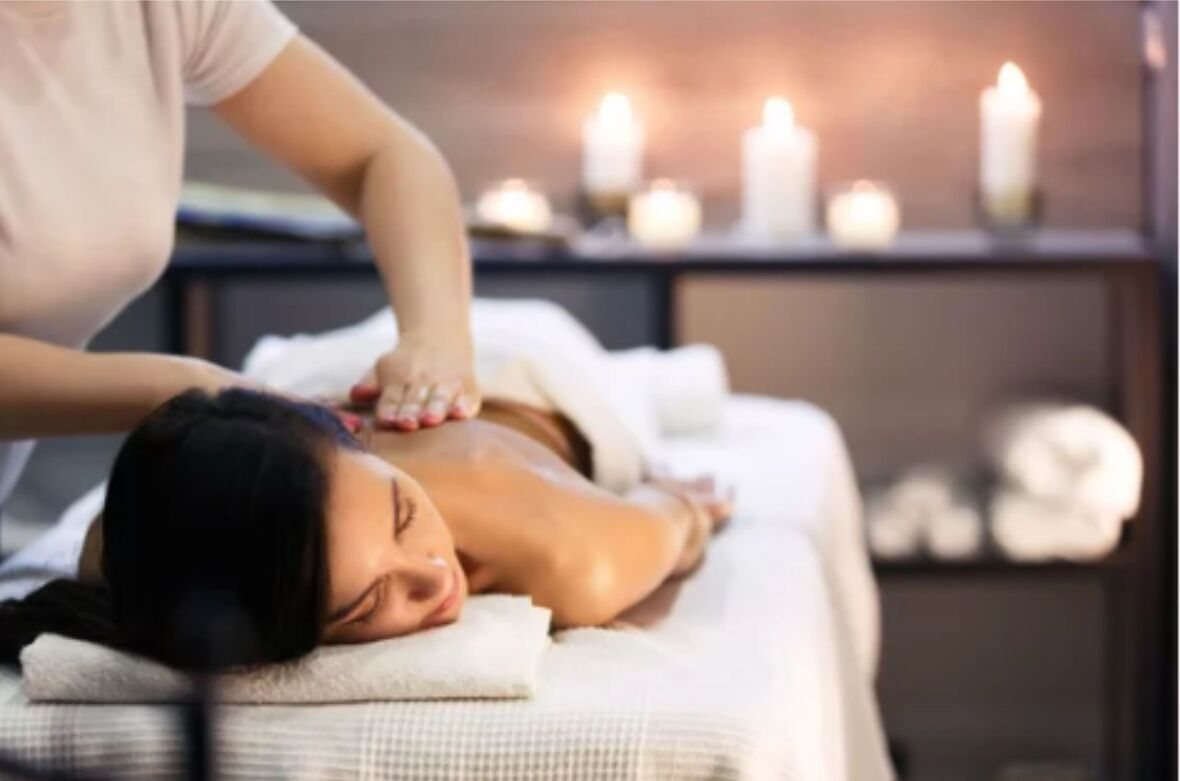September is the start of school, new beginnings, and a great opportunity to grow your practice. We're grateful to know that you're using ethically-sourced products from MTSO for your practice. Our knowledgeable staff is more than happy to assist you with questions you may have.

Looking for upgrades to your clinic or home office? Call us at 780-440-1818 for suggestions on how to update your workspace with an affordable electric table, a sweet aromatherapy program, or new sheets.

MTSO is excited to invite you to a Community Outdoor Concert featuring Thom Golub Quartet on Sunday, September 19. Share the joy with the community, support local musicians, listen to live Jazz, and enjoy spending time with friends - Come out to the concert!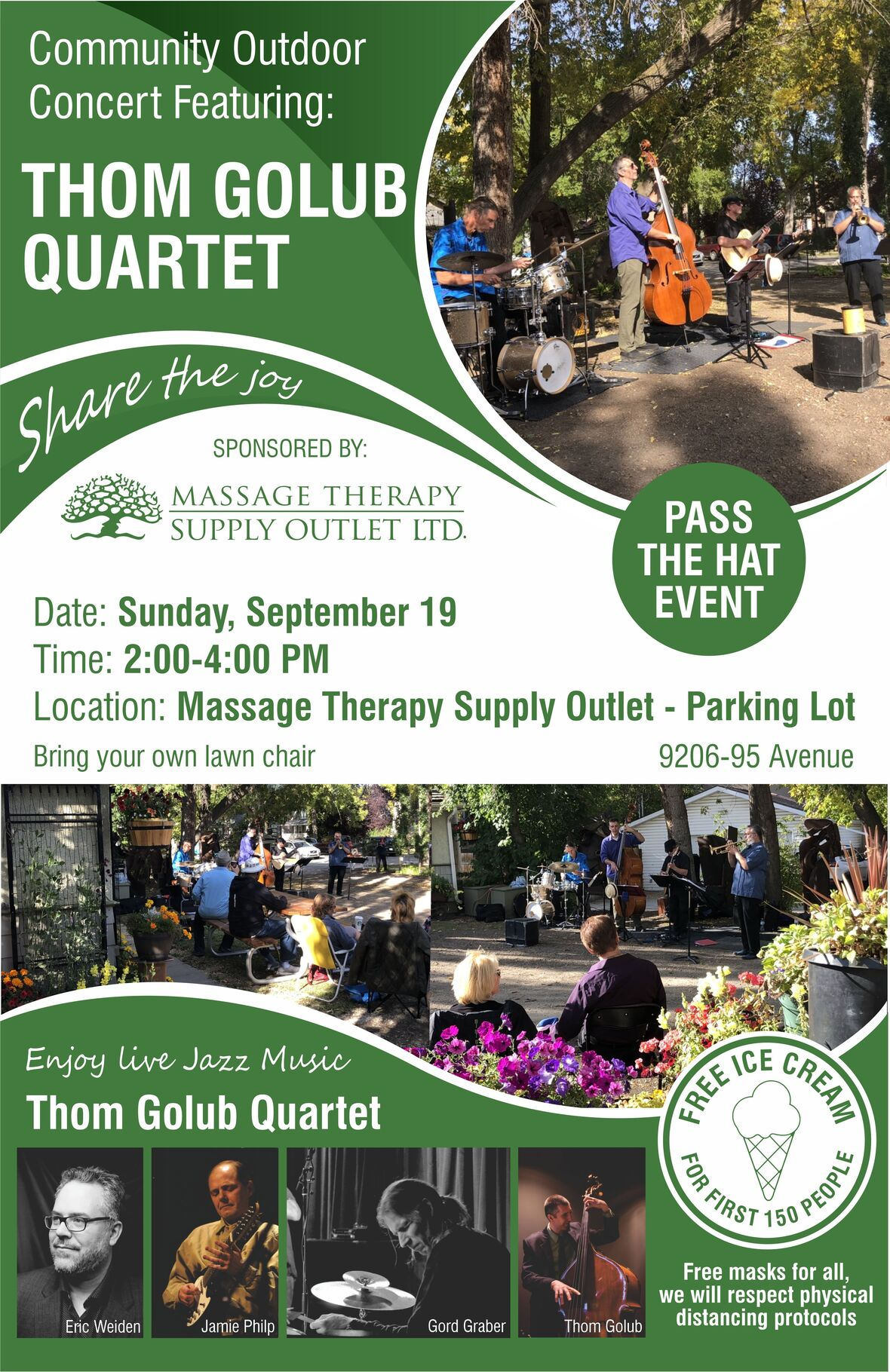table div table+table+table+table+table div table{width:100%;padding:0}table div table+table+table+table+table div table img{width:96.23%;padding:0;float:none}table div table+table+table+table+table div table td{width:100%;padding:0 1.88% 18px}/* styles */# MTSO Making a Difference in Our Community

Joe Clare, owner of MTSO organized a protest on August 18, along with people from the neighborhood in an effort to stop TransEd from planting a narrow strip of grass between the two sidewalks in front of the building. The uneven surface of grass reduces accessibility for people who use wheelchairs and walkers. Many seniors that live across the street from MTSO, walk across the street to enjoy and meet at the MTSO Garden.

"We've watched so many people get hurt here," said Joe Clare, "One lady got hit by a car," he said. Locals have repeatedly brought up accessibility issues with TransEd. Read entire article HERE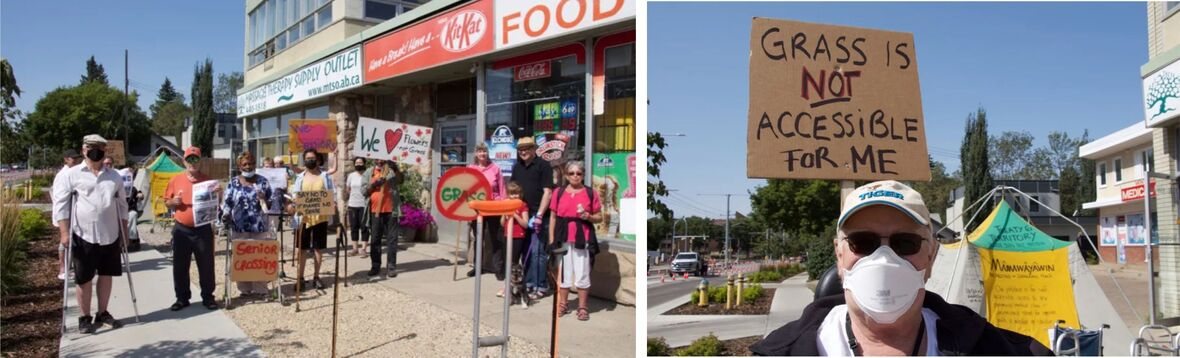Seniors and supporters protest on the northwest corner of 92nd Street and 95th Avenue on Wednesday, Aug. 18. The seniors are trying to prevent TransEd from planting grass between the two sidewalks because they say it is not very accessible for people who use wheelchairs and walkers. (Madeleine Cummings/CBC)
 table div table+table+table+table+table+table+table+table+table div table{width:100%;padding:0}table div table+table+table+table+table+table+table+table+table div table img{width:96.23%;padding:0;float:none}table div table+table+table+table+table+table+table+table+table div table td{width:100%;padding:0 1.88% 18px}/* styles */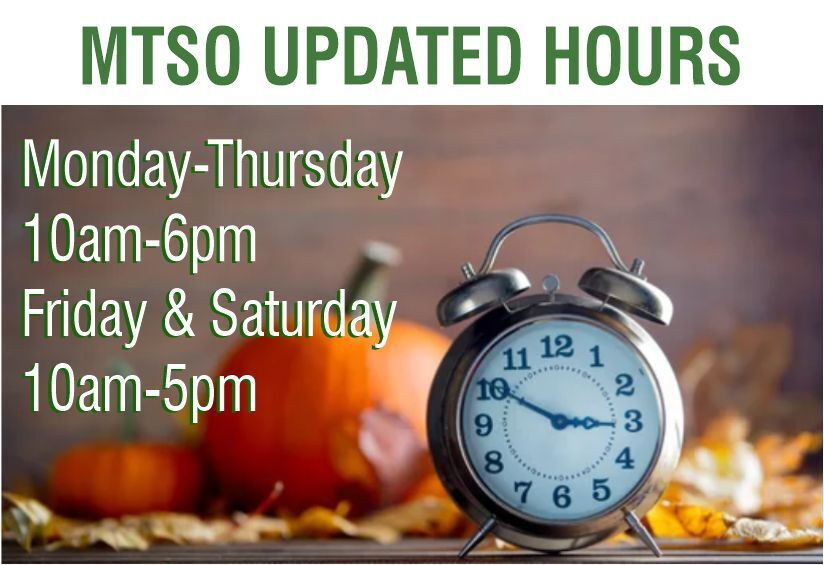table div table+table+table+table+table+table+table+table+table+table+table div table{width:100%;padding:0}table div table+table+table+table+table+table+table+table+table+table+table div table img{width:96.23%;padding:0;float:none}table div table+table+table+table+table+table+table+table+table+table+table div table td{width:100%;padding:0 1.88% 18px}/* styles */# STUDENT PACKAGES

Take advantage of this great pricing to expand your practice with some of our popular must-have products.

All the necessities you need for Massage School, plus a few extras.

These quality student packages are easy on your budget.

▪ Choose either Basic or Deluxe. Both come with Canadian-made portable table and headrest, linens, and accessories.

▪ Add on a Year One or Year Two Student Package for additional sheet sets and other supplies.

 table.module-12{width:91.32%;padding:0}table div table+table+table+table+table+table+table+table+table+table+table+table+table div table{width:91.32%;float:none;margin-left:auto;margin-right:auto;padding:0}table div table+table+table+table+table+table+table+table+table+table+table+table+table div table a{border:0 none;text-decoration:none}table div table+table+table+table+table+table+table+table+table+table+table+table+table div table img{width:100%!important;border:0 none;text-decoration:none}table div table+table+table+table+table+table+table+table+table+table+table+table+table div table td{width:100%;padding:0}/* styles */
 /* styles */
 table div table+table+table+table+table+table+table+table+table+table+table+table+table+table+table+table div table{width:100%;padding:0}table div table+table+table+table+table+table+table+table+table+table+table+table+table+table+table+table div table img{width:96.23%;padding:0;float:none}table div table+table+table+table+table+table+table+table+table+table+table+table+table+table+table+table div table td{width:100%;padding:0 1.88% 18px}/* styles */# PURE CARRIER OILS

Massage Therapy Supply Outlet provides the following pure carrier oils, in a variety of sizes. For use in your massage practice and other uses, we have sourced these natural oils: Jojoba, Almond, Apricot, Coconut, Grapeseed, Rice Bran, Sesame, Fractionated Coconut MCT and Liquid Sunshine blended oil.

 table.module-17{width:92.26%;padding:0}table div table+table+table+table+table+table+table+table+table+table+table+table+table+table+table+table+table+table div table{width:92.26%;float:none;margin-left:auto;margin-right:auto;padding:0}table div table+table+table+table+table+table+table+table+table+table+table+table+table+table+table+table+table+table div table a{border:0 none;text-decoration:none}table div table+table+table+table+table+table+table+table+table+table+table+table+table+table+table+table+table+table div table img{width:100%!important;border:0 none;text-decoration:none}table div table+table+table+table+table+table+table+table+table+table+table+table+table+table+table+table+table+table div table td{width:100%;padding:0}/* styles */
 table div table+table+table+table+table+table+table+table+table+table+table+table+table+table+table+table+table+table+table+table div table{width:100%;padding:0}table div table+table+table+table+table+table+table+table+table+table+table+table+table+table+table+table+table+table+table+table div table img{width:96.23%;padding:0;float:none}table div table+table+table+table+table+table+table+table+table+table+table+table+table+table+table+table+table+table+table+table div table td{width:100%;padding:0 1.88% 18px}/* styles */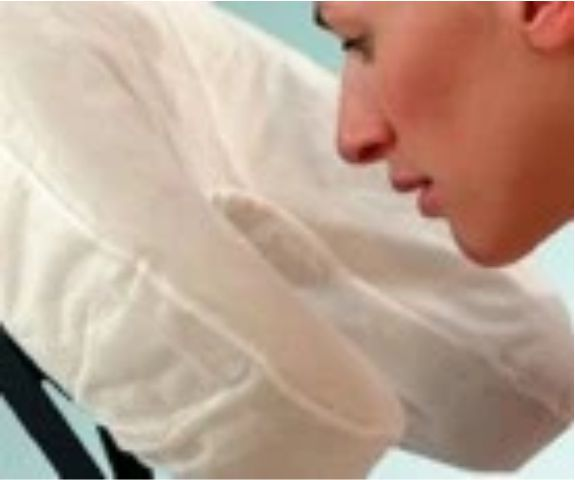Fitted Disposable Face Cradle Covers

# FITTED DISPOSABLE FACE CRADLE COVERS

Made from soft, 25 gsm spunbound fabric, this new and innovative design provides a comfortable, sanitary and disposable barrier for your clients and an easy changeover for between client protection.

▪ Fits all crescent-shaped face rests
▪ Stays put throughout your treatment
▪ Great for table and seated massage
▪ Box of 50
▪ Latex Free

 table div table+table+table+table+table+table+table+table+table+table+table+table+table+table+table+table+table+table+table+table+table+table+table div table{width:100%;padding:0}table div table+table+table+table+table+table+table+table+table+table+table+table+table+table+table+table+table+table+table+table+table+table+table div table img{width:96.23%;padding:0;float:none}table div table+table+table+table+table+table+table+table+table+table+table+table+table+table+table+table+table+table+table+table+table+table+table div table td{width:100%;padding:0 1.88% 18px}/* styles */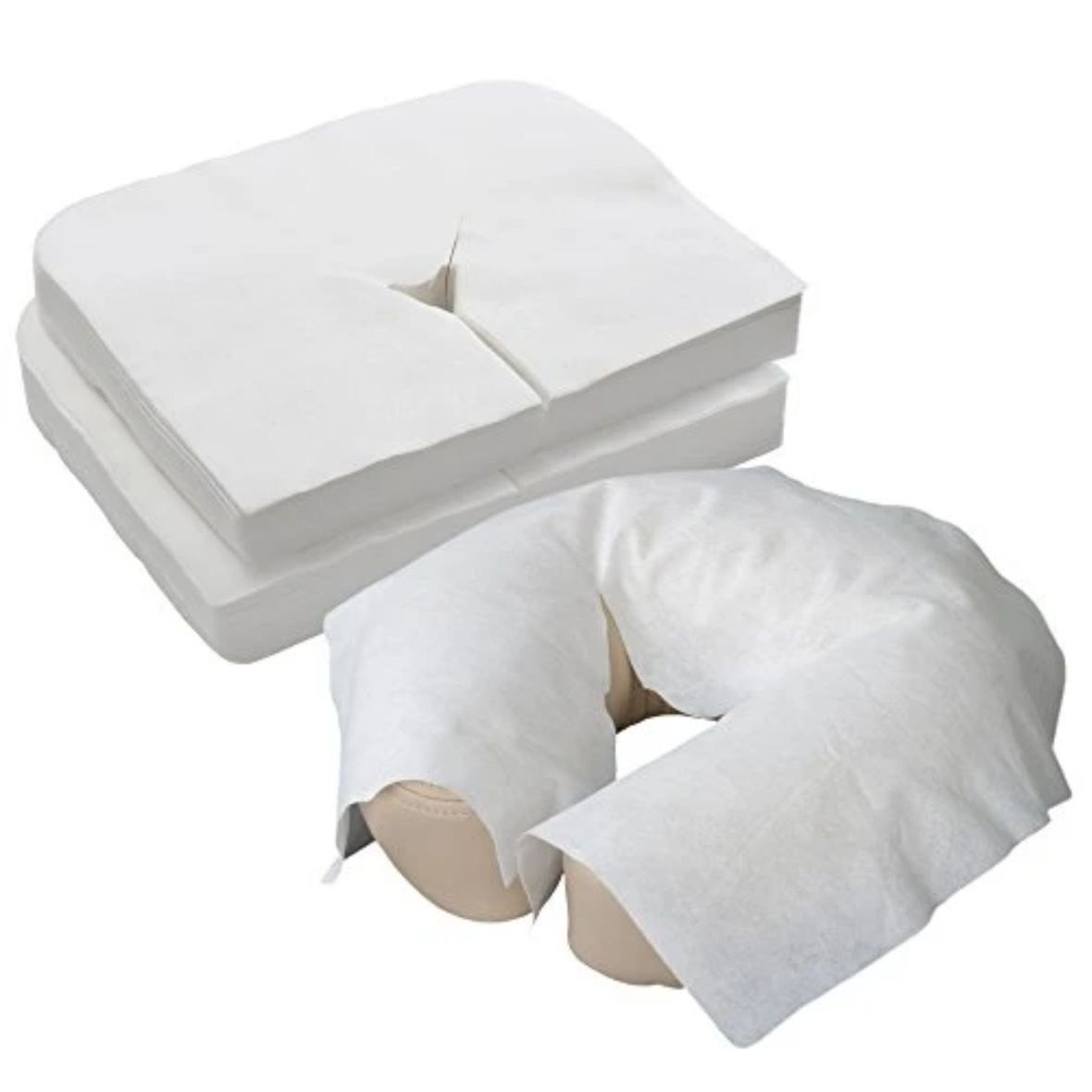Flat Disposable Face Cradle Covers

# FLAT DISPOSABLE FACE CRADLE COVERS

Flat soft disposable face cradle covers package of 100. Product stores easily in table and chair carry cases. Thick, yet soft and silky fabric conforms to the shape of your cushion. Feels like napped cotton. Flat face covers fit almost anything.

 table div table+table+table+table+table+table+table+table+table+table+table+table+table+table+table+table+table+table+table+table+table+table+table+table+table+table div table{width:100%;padding:0}table div table+table+table+table+table+table+table+table+table+table+table+table+table+table+table+table+table+table+table+table+table+table+table+table+table+table div table img{width:96.23%;padding:0;float:none}table div table+table+table+table+table+table+table+table+table+table+table+table+table+table+table+table+table+table+table+table+table+table+table+table+table+table div table td{width:100%;padding:0 1.88% 18px}/* styles */table.module-26{width:95.85%;padding:0}table div table+table+table+table+table+table+table+table+table+table+table+table+table+table+table+table+table+table+table+table+table+table+table+table+table+table+table div table{width:95.85%;float:none;margin-left:auto;margin-right:auto;padding:0}table div table+table+table+table+table+table+table+table+table+table+table+table+table+table+table+table+table+table+table+table+table+table+table+table+table+table+table div table a{border:0 none;text-decoration:none}table div table+table+table+table+table+table+table+table+table+table+table+table+table+table+table+table+table+table+table+table+table+table+table+table+table+table+table div table img{width:100%!important;border:0 none;text-decoration:none}table div table+table+table+table+table+table+table+table+table+table+table+table+table+table+table+table+table+table+table+table+table+table+table+table+table+table+table div table td{width:100%;padding:0}/* styles */
 table div table+table+table+table+table+table+table+table+table+table+table+table+table+table+table+table+table+table+table+table+table+table+table+table+table+table+table+table+table div table{width:100%;padding:0}table div table+table+table+table+table+table+table+table+table+table+table+table+table+table+table+table+table+table+table+table+table+table+table+table+table+table+table+table+table div table img{width:96.23%;padding:0;float:none}table div table+table+table+table+table+table+table+table+table+table+table+table+table+table+table+table+table+table+table+table+table+table+table+table+table+table+table+table+table div table td{width:100%;padding:0 1.88% 18px}/* styles */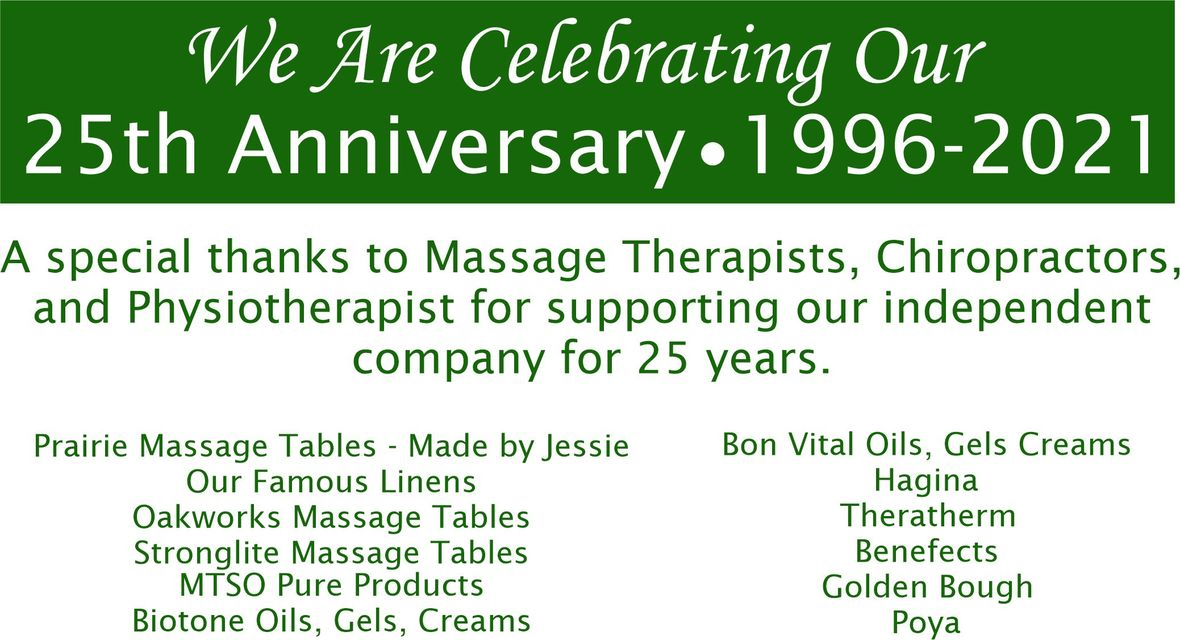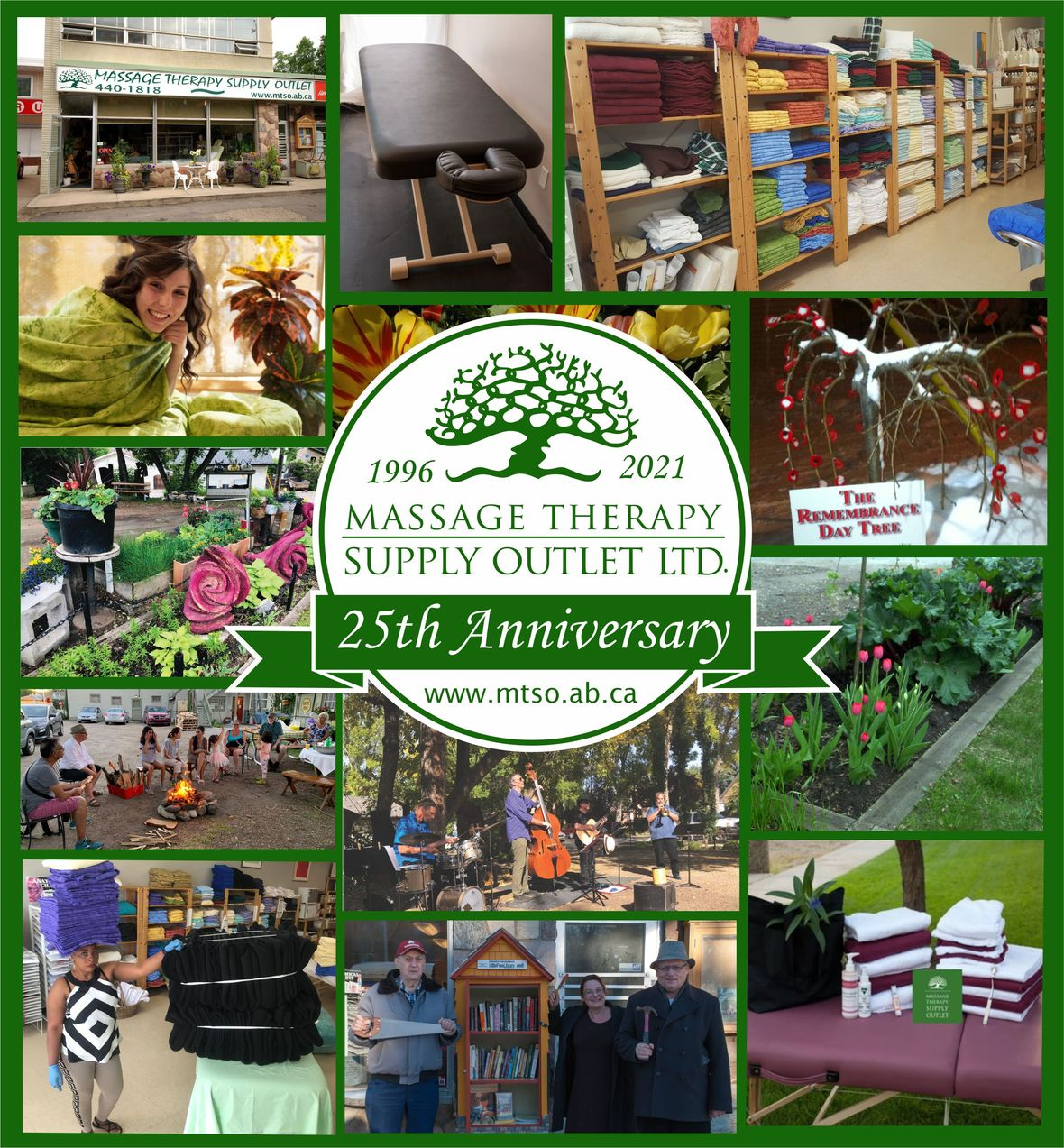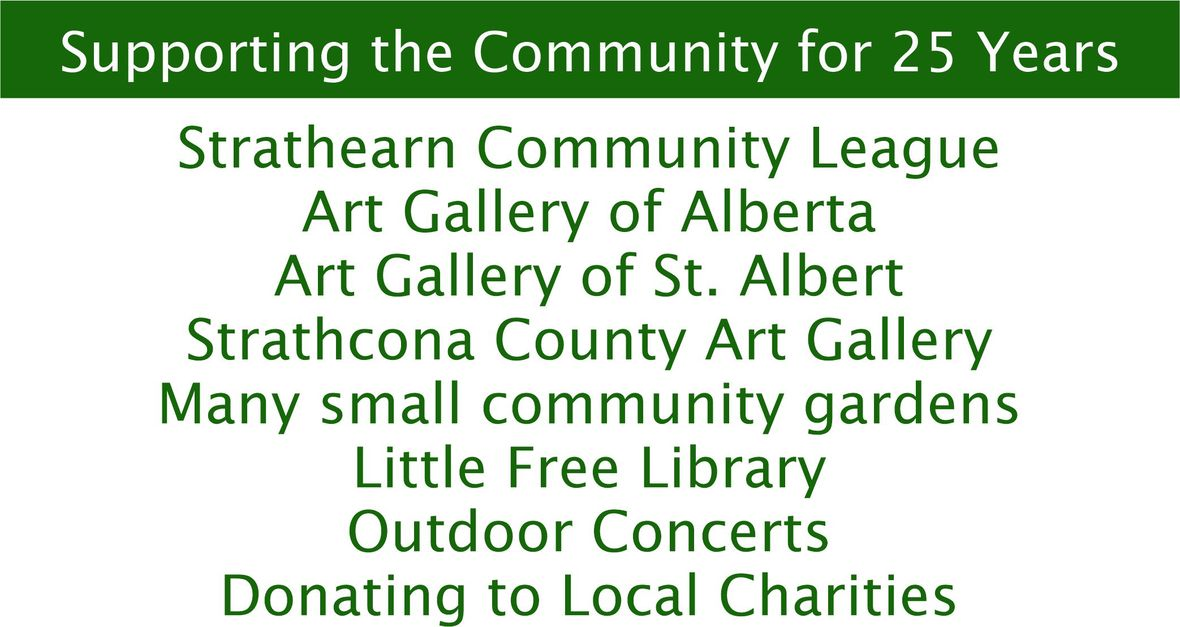table div table+table+table+table+table+table+table+table+table+table+table+table+table+table+table+table+table+table+table+table+table+table+table+table+table+table+table+table+table+table+table+table+table div table{width:100%;padding:0}table div table+table+table+table+table+table+table+table+table+table+table+table+table+table+table+table+table+table+table+table+table+table+table+table+table+table+table+table+table+table+table+table+table div table img{width:96.23%;padding:0;float:none}table div table+table+table+table+table+table+table+table+table+table+table+table+table+table+table+table+table+table+table+table+table+table+table+table+table+table+table+table+table+table+table+table+table div table td{width:100%;padding:0 1.88% 18px}/* styles */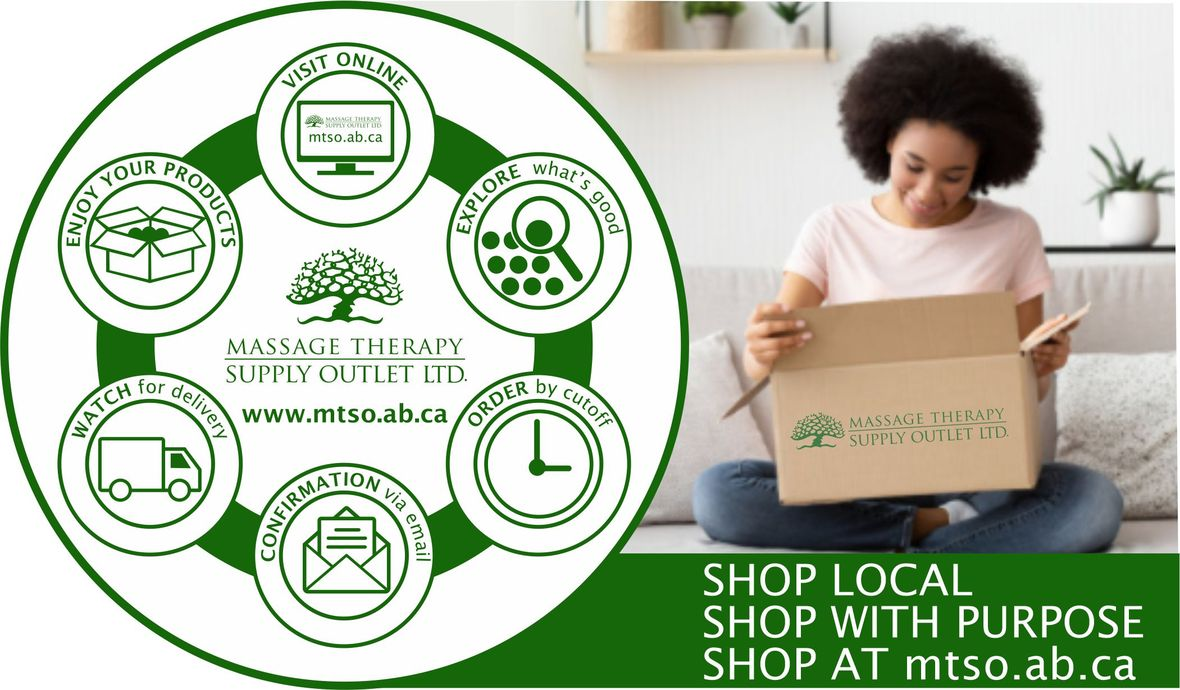# CURBSIDE PICKUP IS AVAILABLE

In addition to in-store shopping and shipping, curbside pick-up is also available. You can either phone-in your order or place order online. When placing your order online choose Local Pick Up at check out. We will contact you when your order is ready.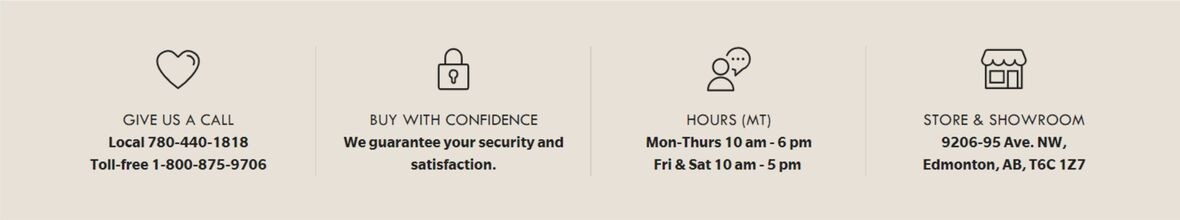/* styles */ Browse and buy from the extensive collection of quality products at our online store, always open, 24/7/365. Drop by our Edmonton store and showroom: : Mon-Fri: 10:00-6:00, Sat: 10:00-5:00
 Like   Tweet   Pin   in